# Chapter 14 Customizing/Polishing Graphics

library(tidyverse)   # loading ggplot2 and dplyr
library(patchwork)   # arranging multiple graphs into 1 figure
library(viridis)     # The viridis color schemes
library(latex2exp)   # For plotting math notation
library(plotly)     # for interactive hover-text

I have a YouTube Video Lecture for this chapter.

We have already seen how to create many basic graphs using the ggplot2 package. However we haven’t addressed many common scenarios. In this chapter we cover many graphing tasks that occur.

## 14.1 Multi-plots

There are several cases where it is reasonable to need to take several possibly unrelated graphs and put them together into a single larger graph. This is not possible using facet_wrap or facet_grid as they are intended to make multiple highly related graphs. We could get into the details of how ggplot2 works using the grid system, but I find looking to other packages that have already done this to be helpful. The examples below are not the only packages that do this, but rather are just two solutions I’ve used in the past. Other solutions include the ggpubr and patchwork packages.

### 14.1.1cowplot package

Claus O. Wilke wrote a lovely book about data visualization and also wrote an R package to help him tweek his plots. One of the functions in his cowplot package is called plot_grid and it takes in any number of plots and lays them out on a grid.

P1 <- ggplot(iris, aes(x=Sepal.Length, y=Sepal.Width, color=Species)) +
geom_point(size=3)  + labs(title='P1 - Iris')
P2 <- ggplot(trees, aes(x=Height, y=Volume, color=Girth)) +
geom_point()  + labs(title='P2 - Cherry Trees')
P3 <- ggplot(iris, aes(x=Sepal.Length)) +
geom_histogram(bins=30) + labs(title='P3 - Iris')
P4 <- ggplot(iris, aes(x=Species, y=Sepal.Length, fill=Species)) +
geom_boxplot() + labs(title='P4 - Iris')

cowplot::plot_grid(P1, P2, P3, P4)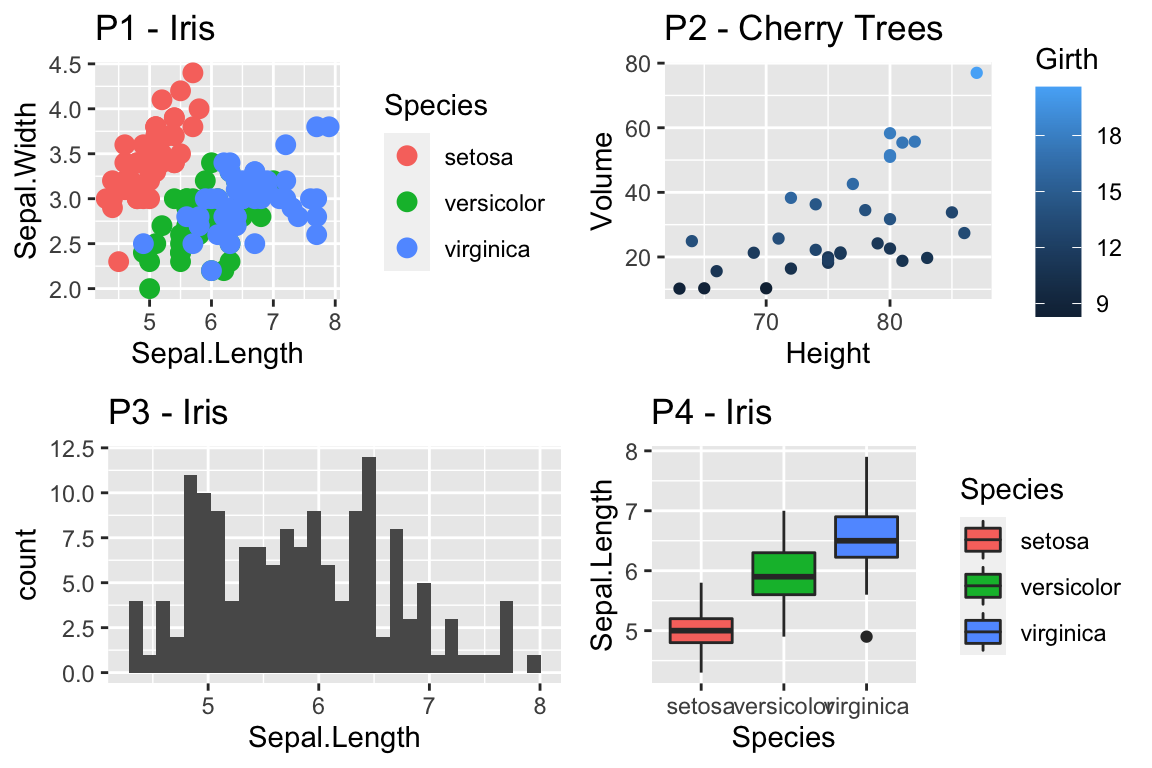Notice that the graphs are by default are arranged in a 2x2 grid. We could adjust the number or rows/columns using the nrow and ncol arguments. Furthermore, we could add labels to each graph so that the figure caption to refer to “Panel A” or “Panel B” as appropriate using the labels option.

cowplot::plot_grid(P2, P3, P4, nrow=1, labels=c('A','B','C'))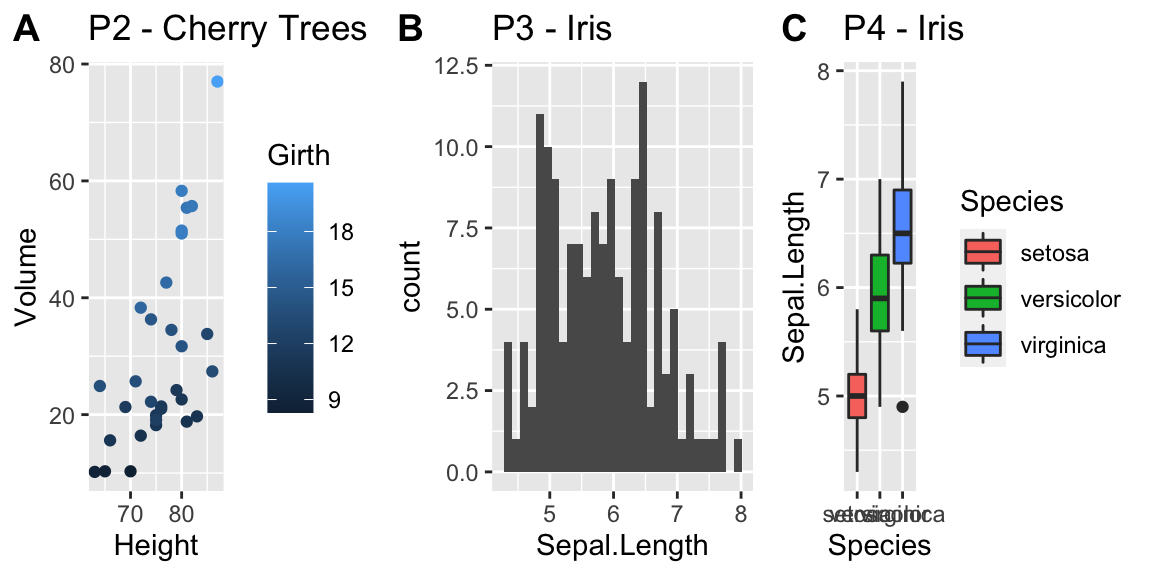### 14.1.2patchwork package

This package is quite powerful and is written by one of the maintainers of ggplot2. After working with this package, it is quite a bit more powerful than cowplot and far more flexible.

The package documentation gets into far more detail than we will in the following examples.

# Just adding plots defaults to equally sized grids
P1 + P2 + P3 + P4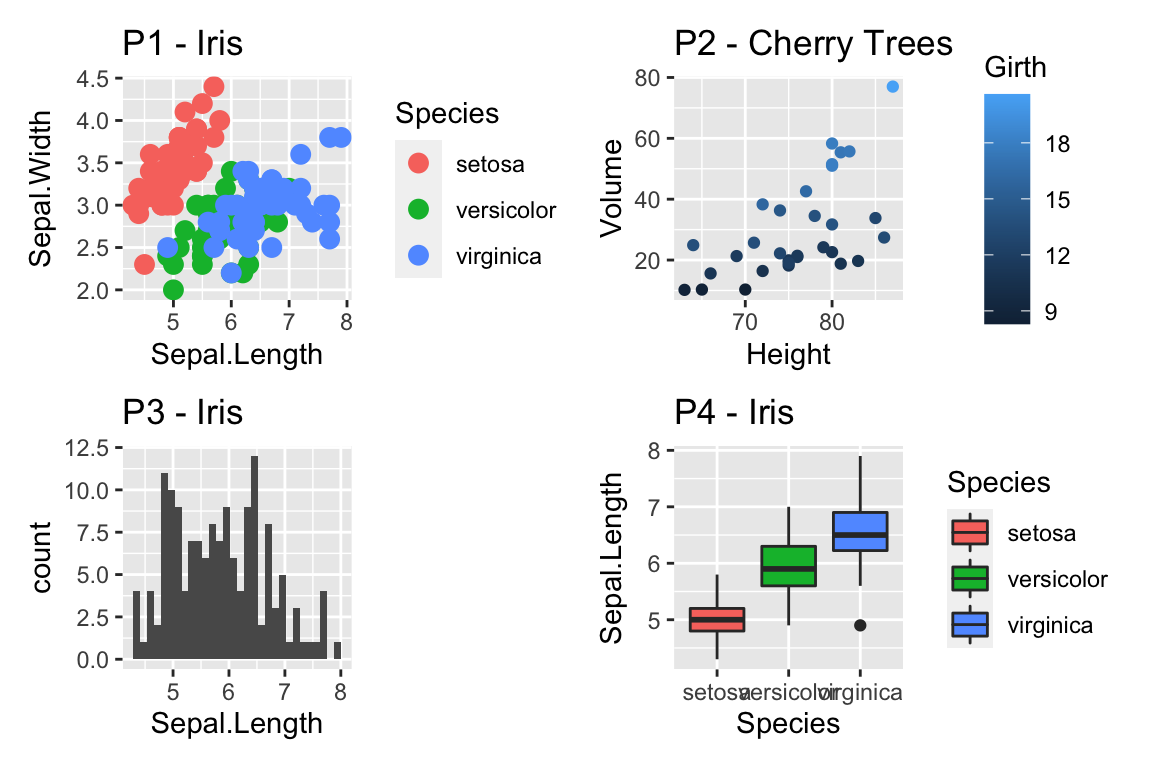# grouping with parentheses. This induces a hierarchy of the graph
# Notice the amount of space allocated is set to be equal among the
# groups at the same level of the hierarchy.

# division produces new rows.
(P1 | P2) / P3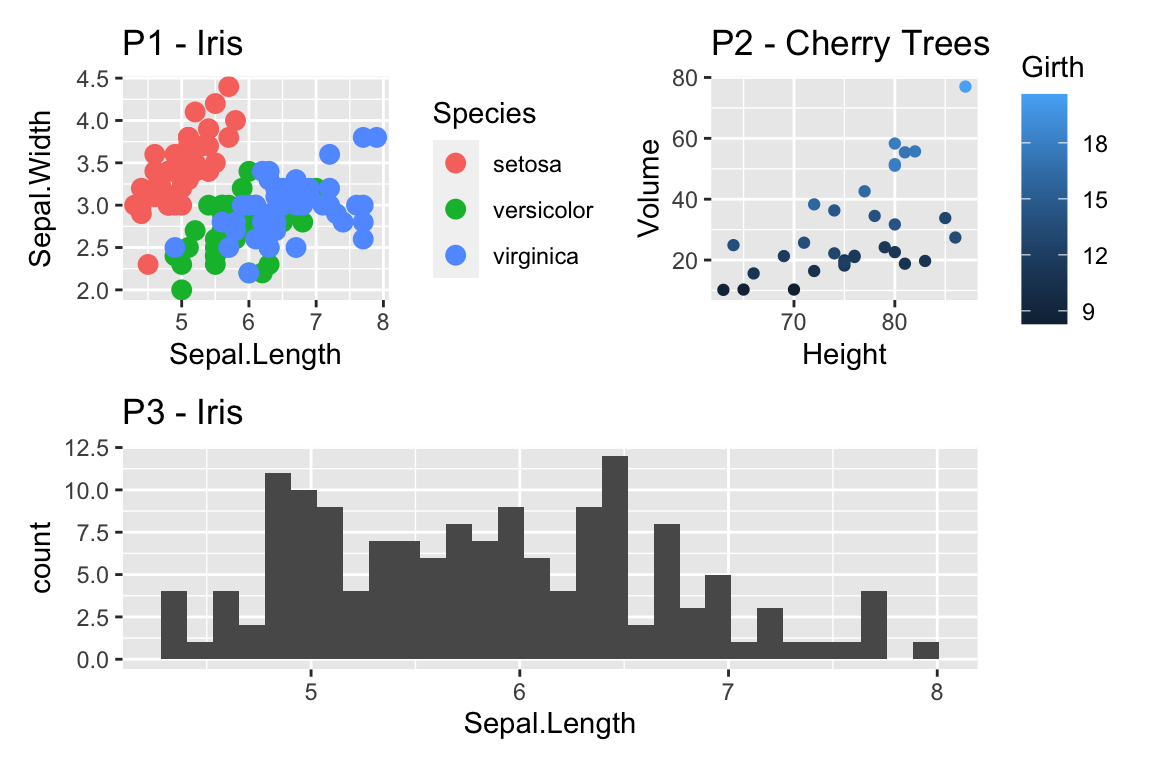# A bar produces a new column
(P1 + P2) | P3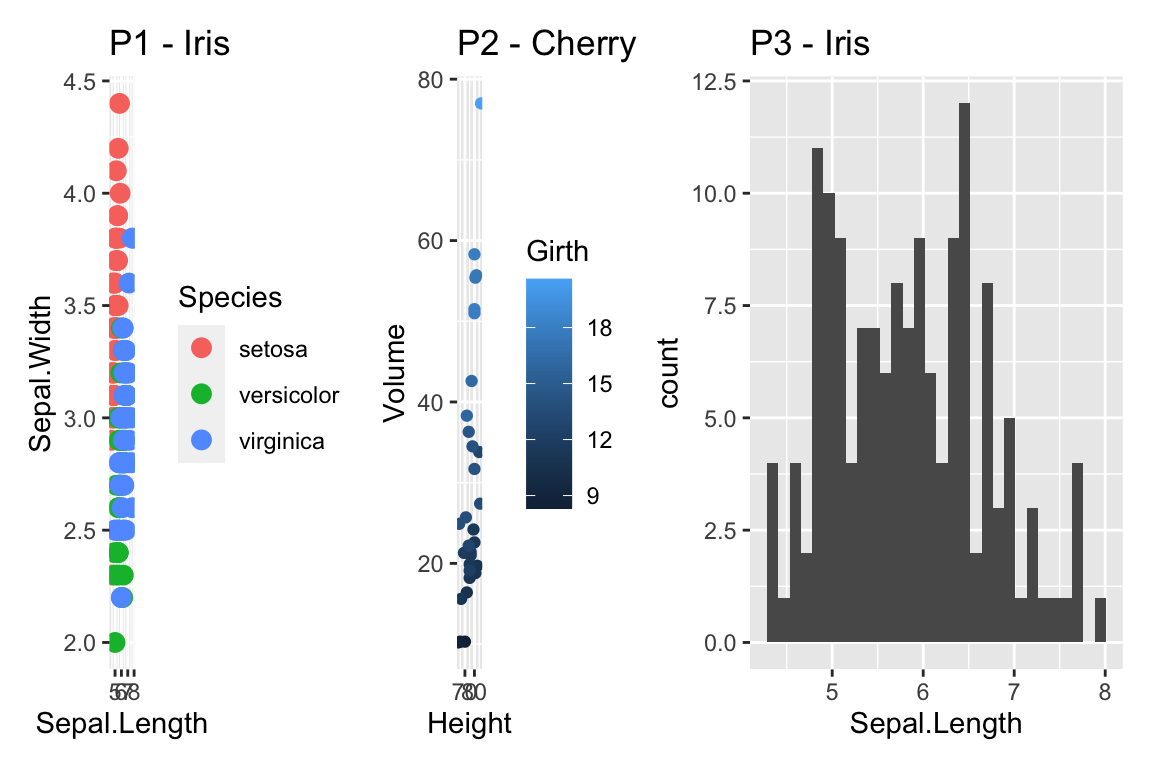# We can add a title to the composite graph as well as add
# panel identifiers post-hoc. Notice this has to apply to
# the top level of the hierarchy.
(P1 | (P2/P4)) +
plot_annotation(
title = 'Composite Graph Title',
subtitle = 'With a compelling subtitle',
caption = 'Iris Data from Edgar Anderson (1935)'
)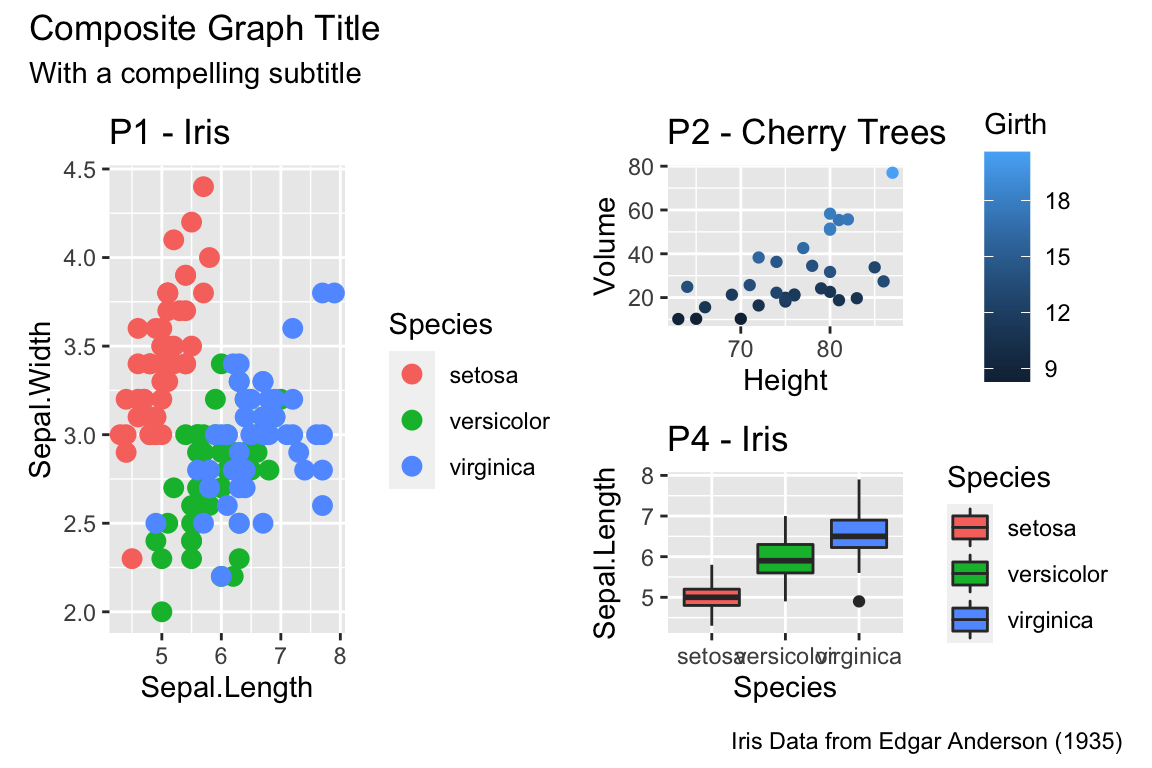The last trick is to recognize that often we have the same legends in the graph and it would be nice to collect them all into one spot. Lets collect them all into one area.

(P1 | (P2/P4)) +
plot_annotation(title = 'Composite Graph Title') +
plot_layout(guides = 'collect')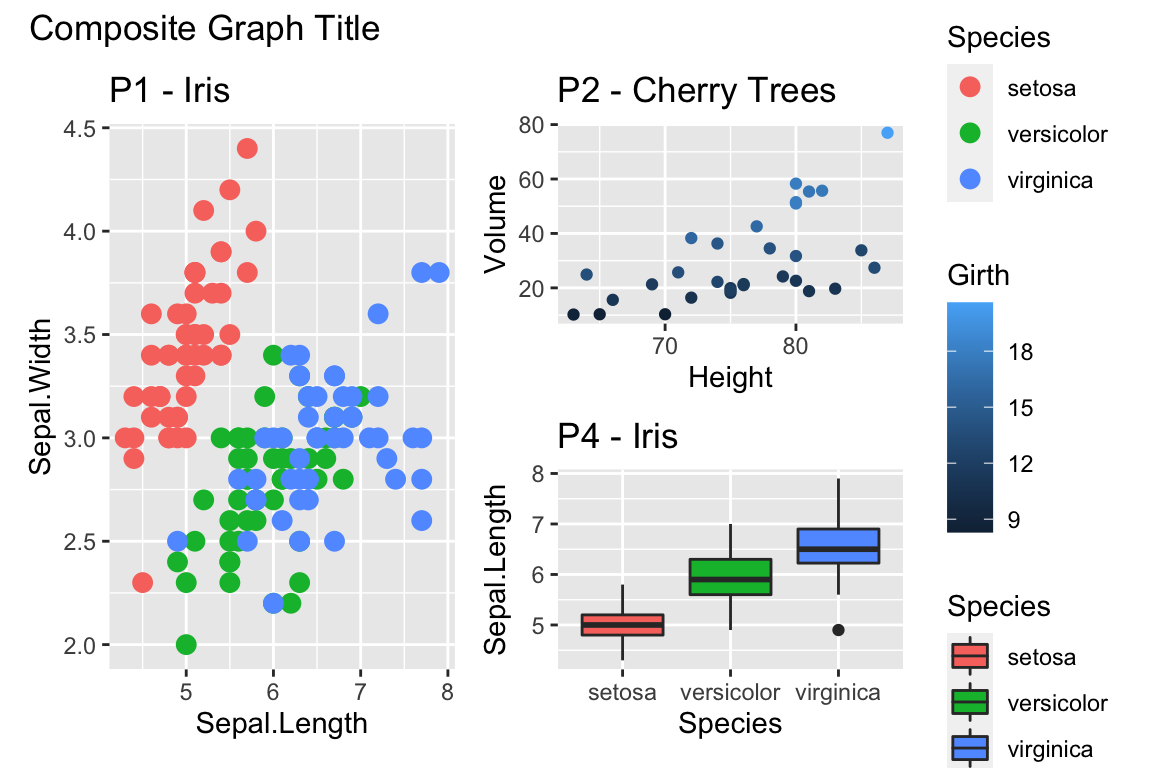Notice we have a fill guide and a color guide. They are different guides as far as ggplot2 is concerned, but it would be nice to remove one of them.

((P1 + theme(legend.position = 'none')) | (P2/P4)) +
plot_annotation(
title = 'Composite Graph Title',
subtitle = 'With a compelling subtitle',
caption = 'Iris Data from Edgar Anderson (1935)') +
plot_layout(guides = 'collect')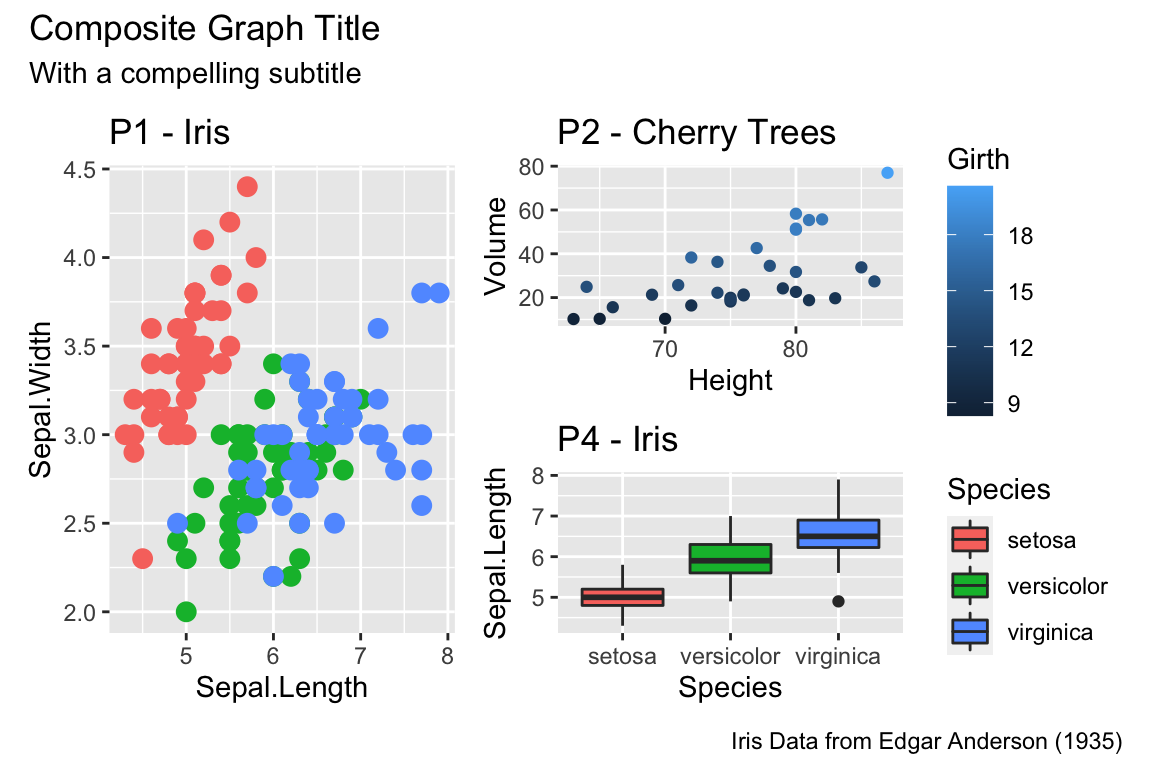## 14.2 Customized Scales

While ggplot typically produces very reasonable choices for values for the axis scales and color choices for the color and fill options, we often want to tweak them.

### 14.2.1 Color Scales

#### 14.2.1.1 Manually Select Colors

For an individual graph, we might want to set the color manually. Within ggplot there are a number of scale_XXX_ functions where the XXX is either color or fill.

cowplot::plot_grid(
P1 + scale_color_manual( values=c('red','navy','forest green') ),
P2 + scale_color_gradient(low = 'blue', high='red')
)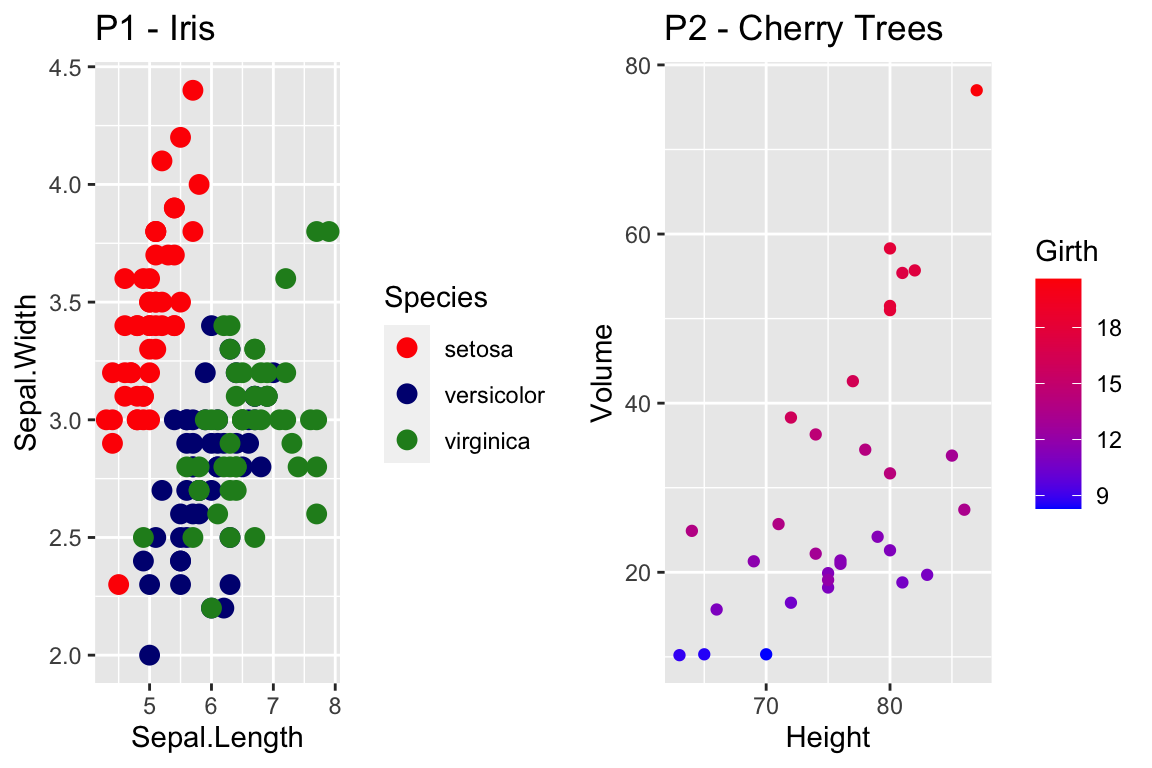For continuous scales for fill and color, there is also a scale_XXX_gradient2() function which results in a divergent scale where you set the low and high values as well as the midpoint color and value. There is also a scale_XXX_grandientn() function that allows you to set as many colors as you like to move between.

cowplot::plot_grid(
P2 + scale_color_gradient2( low = 'black', mid='white', midpoint=14, high='red' ),
P2 + scale_color_gradientn(colors = c('red','orange','yellow','green','blue','violet') )
)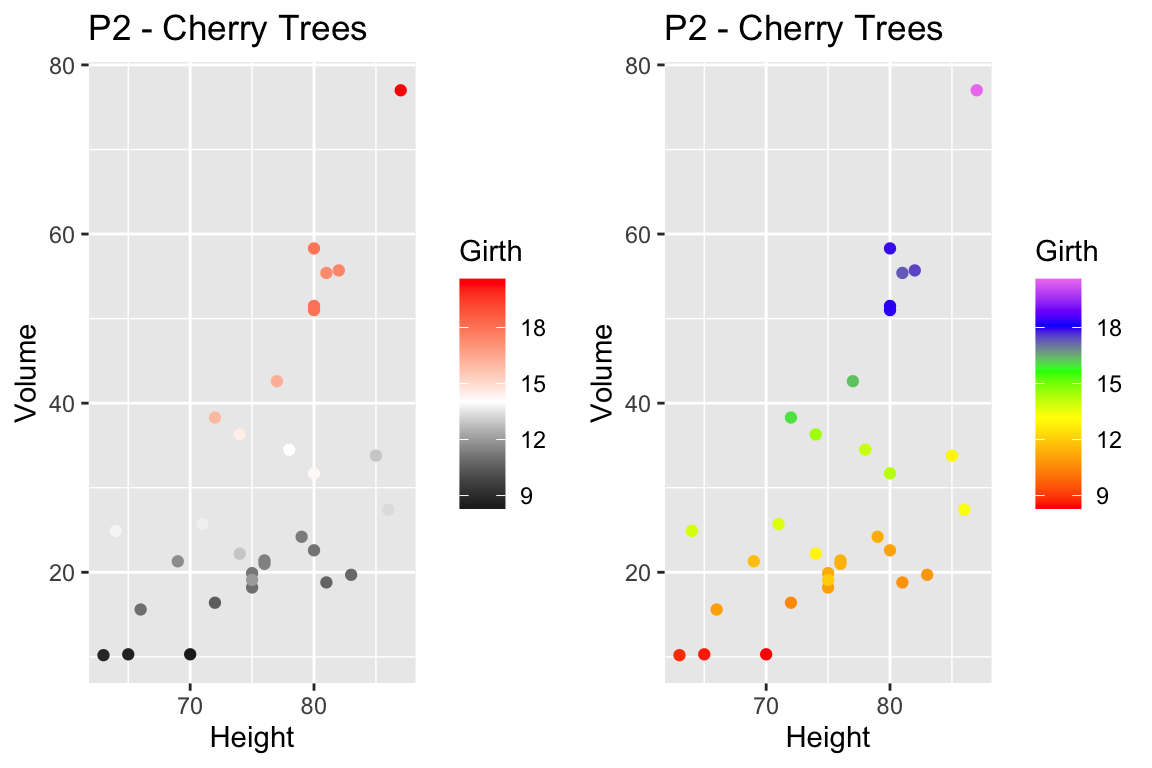Generally I find that I make poor choices when picking colors manually, but there are times that it is appropriate.

#### 14.2.1.2 Palettes

In choosing color schemes, a good approach is to use a color palette that has already been created by folks that know about how colors are displayed and what sort of color blindness is possible. There are two palette options that we’ll discuss, but there are a variety of other palettes available by downloading a package.

##### 14.2.1.2.1RColorBrewer palettes

Using the ggplot::scale_XXX_brewer() functions, we can easily work with the package RColorBrewer which provides a nice set of color palettes. These palettes are separated by purpose.

Qualitative palettes employ different hues to create visual differences between classes. These palettes are suggested for nominal or categorical data sets.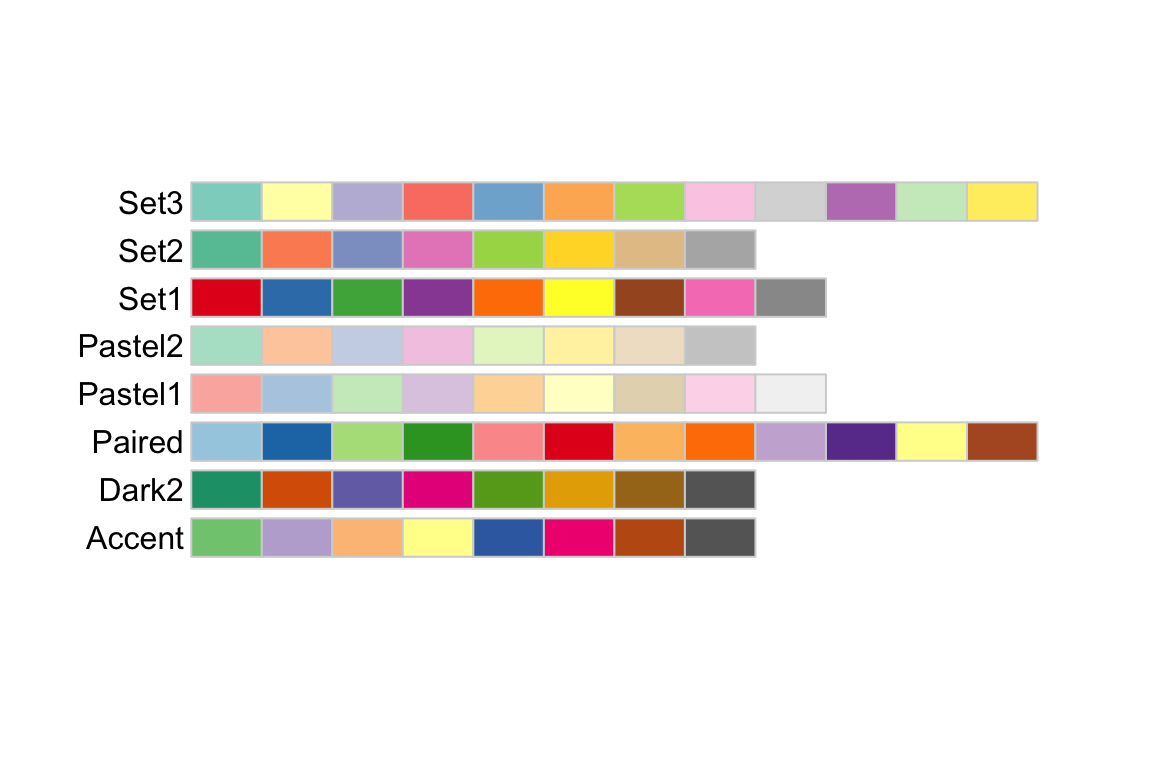Sequential palettes progress from light to dark. When used with interval data, light colors represent low data values and dark colors represent high data values.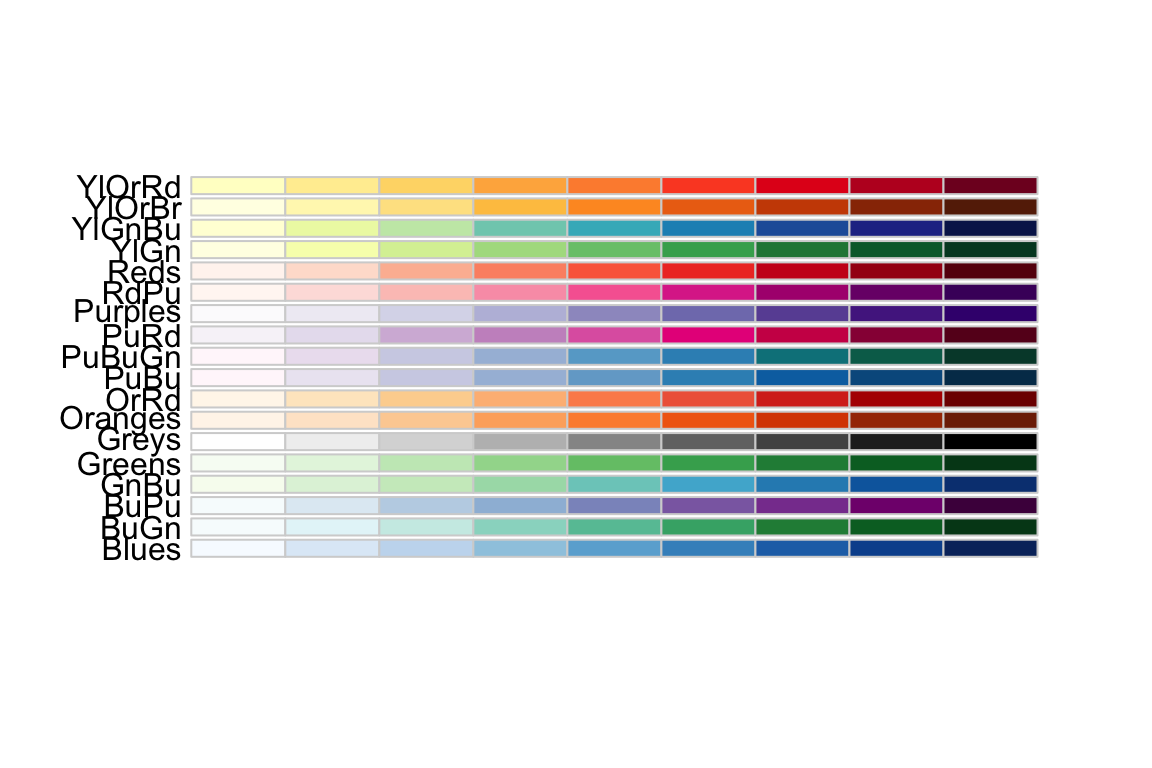Diverging palettes are composed of darker colors of contrasting hues on the high and low extremes and lighter colors in the middle.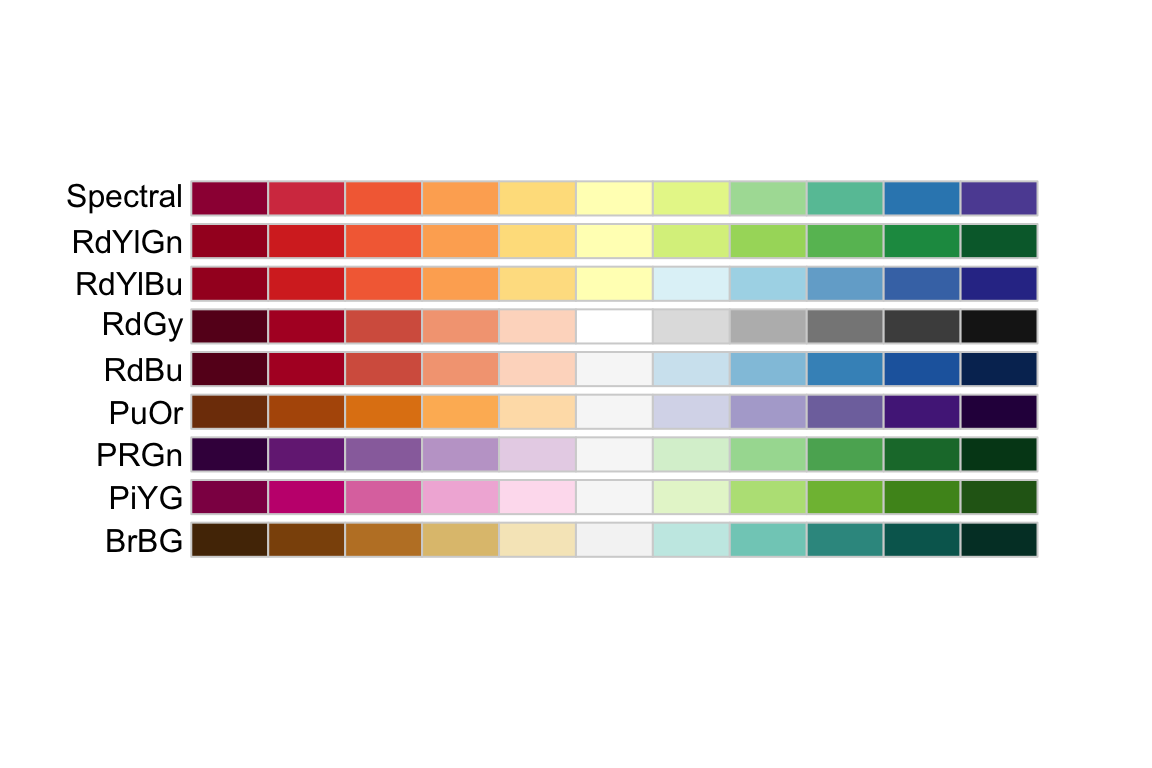To use one of these palettes, we just need to pass the palette name to scale_color_brewer or scale_fill_brewer

cowplot::plot_grid(
P1 + scale_color_brewer(palette='Dark2'),
P4 + scale_fill_brewer(palette='Dark2')
)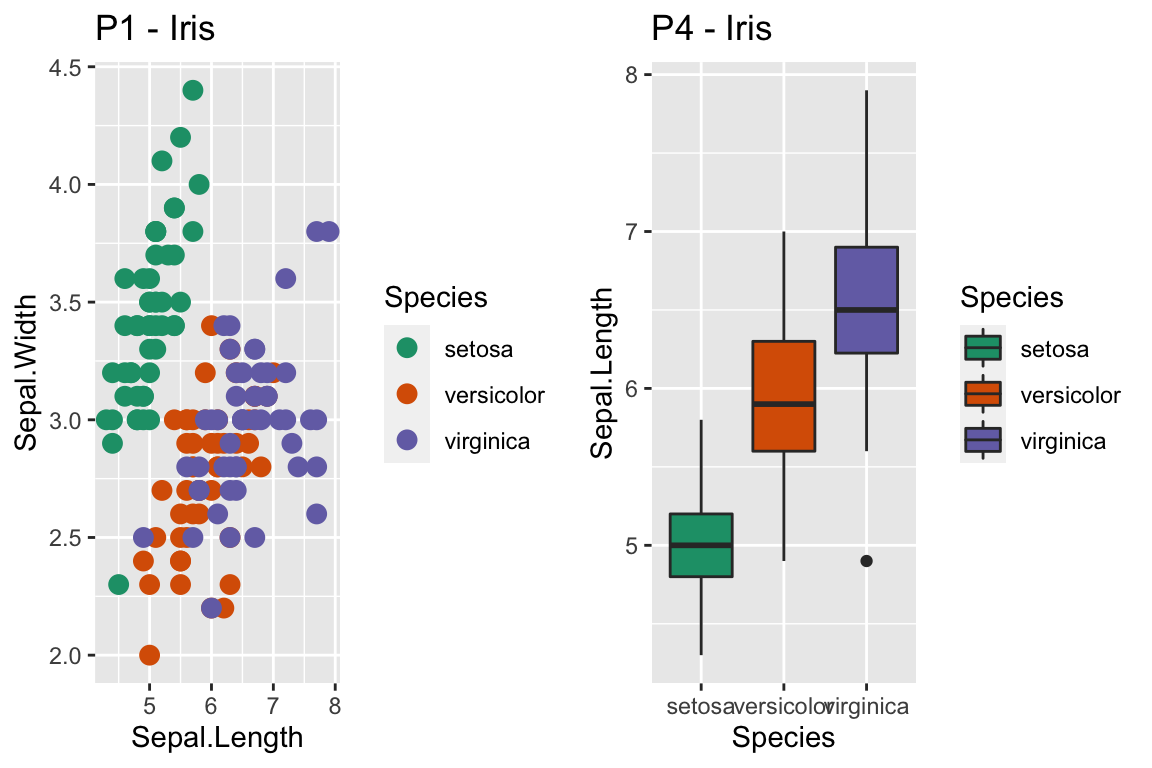##### 14.2.1.2.2viridis palettes

The package viridis sets up a few different color palettes that have been well thought out and maintain contrast for people with a variety of color-blindess types as well as being converted to grey-scale.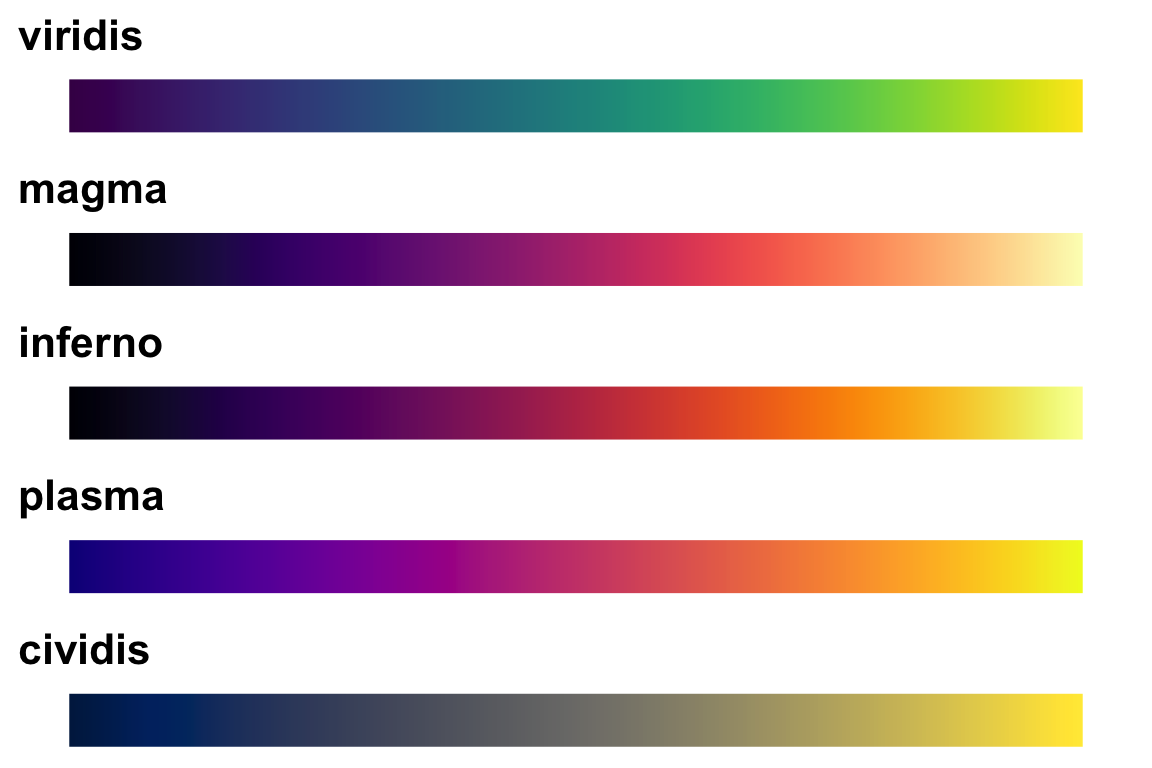cowplot::plot_grid(
P1 + scale_color_viridis_d(option='plasma'),      # _d for discrete
P2 + scale_color_viridis_c( option='viridis') )   # _c for continuous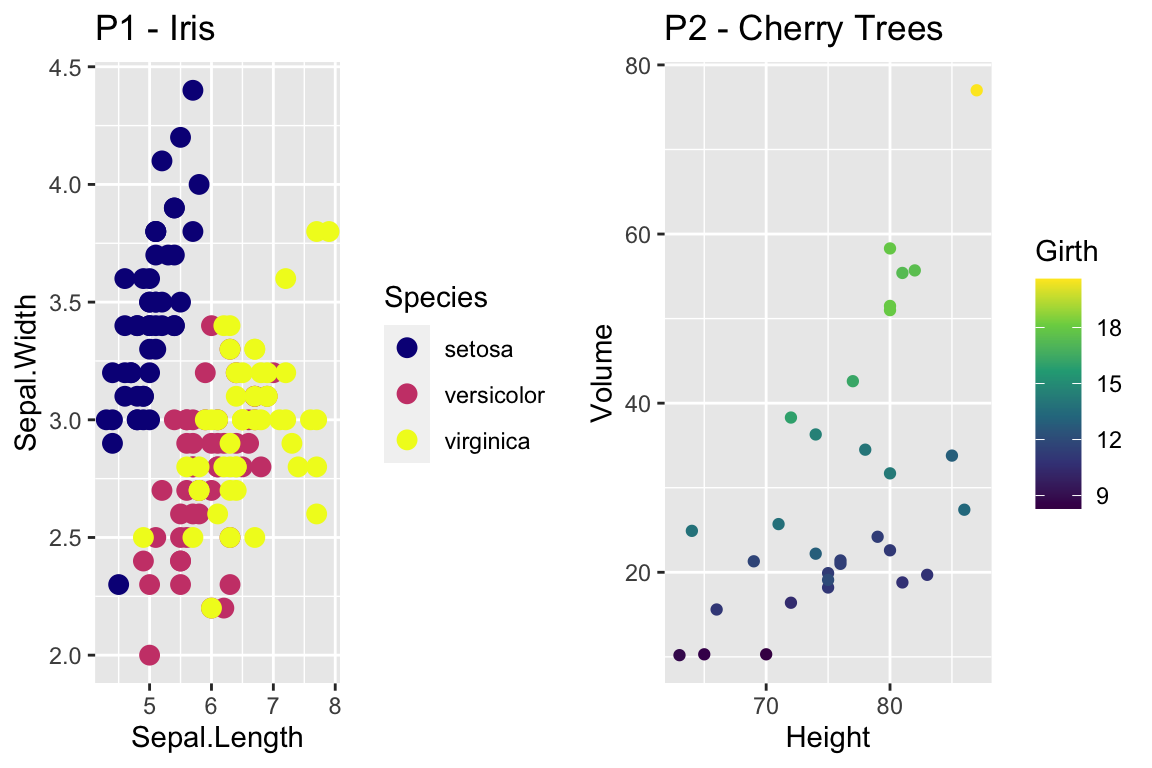There are a bunch of other packages that manage color palettes such as paletteer, ggsci and wesanderson.

### 14.2.2 Setting major & minor ticks

For continuous variables, we need to be able to control what tick and grid lines are displayed. In ggplot, there are major and minor breaks and the major breaks are labeled and minor breaks are in-between the major breaks. The break point labels can also be set.

ggplot(trees, aes(x=Height, y=Volume)) + geom_point() +
scale_x_continuous( breaks=seq(65,90, by=5), minor_breaks=65:90 ) +
scale_y_continuous( breaks=c(30,50), labels=c('small','big') )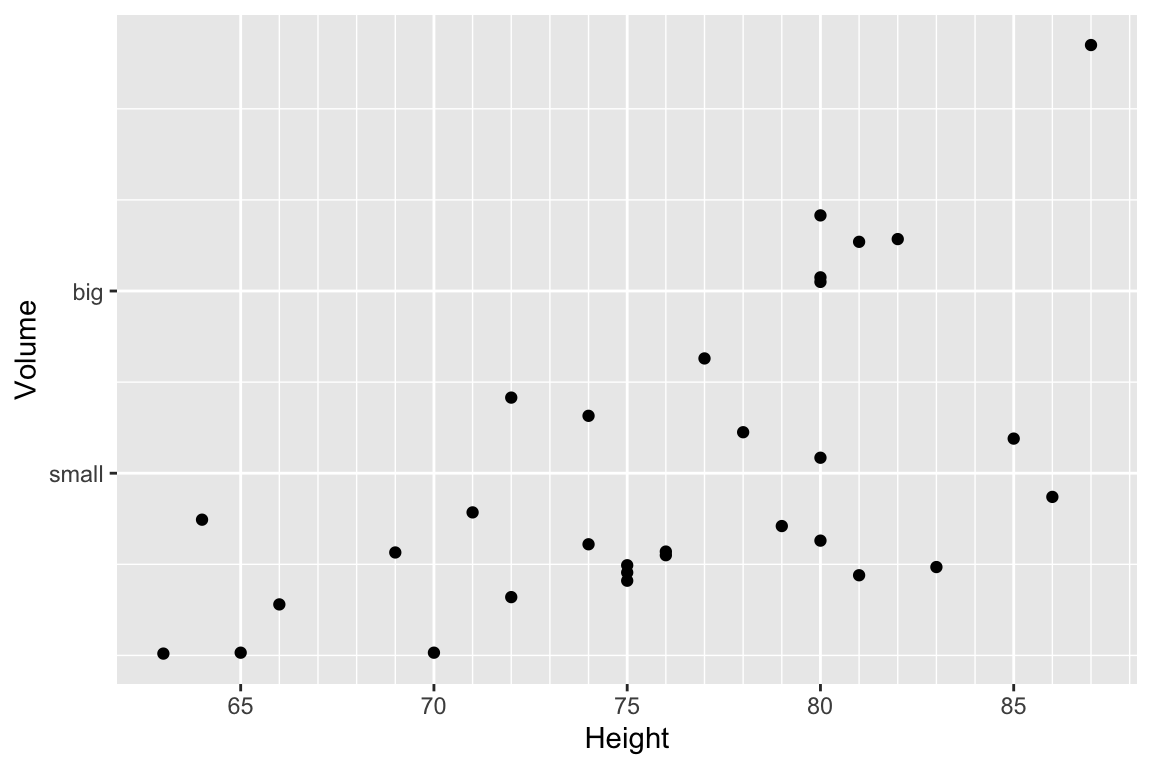### 14.2.3 Log Scales

For this example, we’ll use the ACS data from the Lock5Data package that has information about Income (in thousands of dollars) and Age. Lets make a scatter plot of the data.

# Import the data and drop any zeros
data('ACS', package='Lock5Data')
ACS <- ACS %>%
drop_na() %>% filter(Income > 0)

cowplot::plot_grid(
ggplot(ACS, aes(x=Age, y=Income)) +
geom_point(),
ggplot(ACS, aes(x=Age, y=log10(Income))) +
geom_point()
)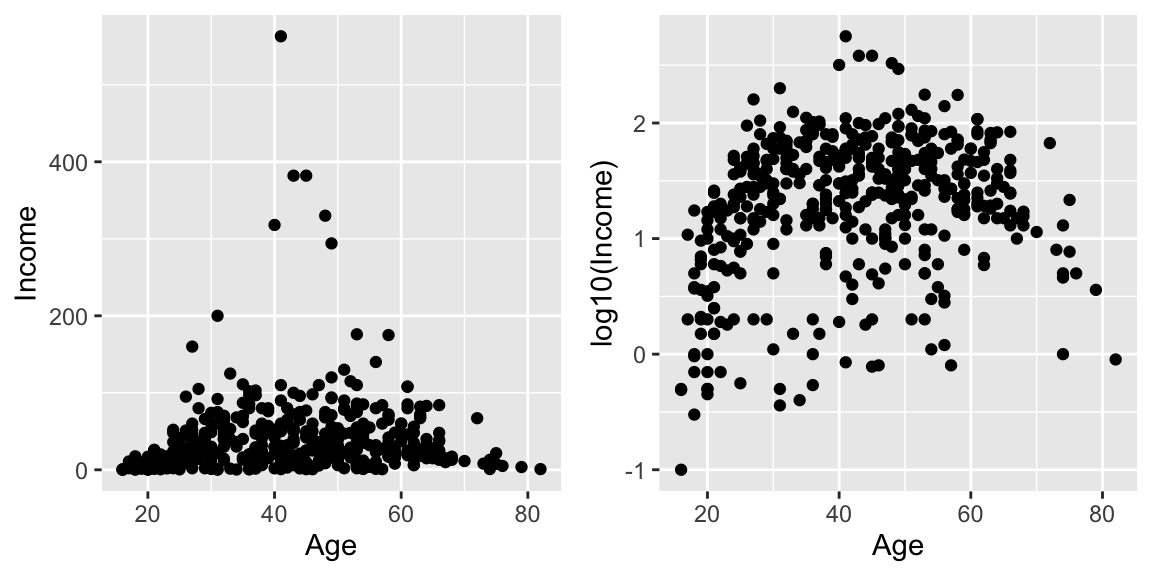Plotting the raw data results in an ugly graph because six observations dominate the graph and the bulk of the data (income < $100,000) is squished together. One solution is to plot income on the $$\log_{10}$$ scale. The second graph does that, but the labeling is all done on the log-scale and most people have a hard time thinking in terms of logs. This works quite well to see the trend of peak earning happening in a persons 40s and 50s, but the scale is difficult for me to understand (what does $$\log_{10}\left(X\right)=1$$ mean here? Oh right, that is $$10^{1}=X$$ so that is the$10,000 line). It would be really nice if we could do the transformation but have the labels on the original scale.

cowplot::plot_grid(
ggplot(ACS, aes(x=Age, y=Income)) +
geom_point() +
scale_y_log10(),
ggplot(ACS, aes(x=Age, y=Income)) +
geom_point() +
scale_y_log10(breaks=c(1,10,100),
minor=c(1:10,
seq( 10, 100,by=10 ),
seq(100,1000,by=100))) +
ylab('Income (1000s of dollars)')
)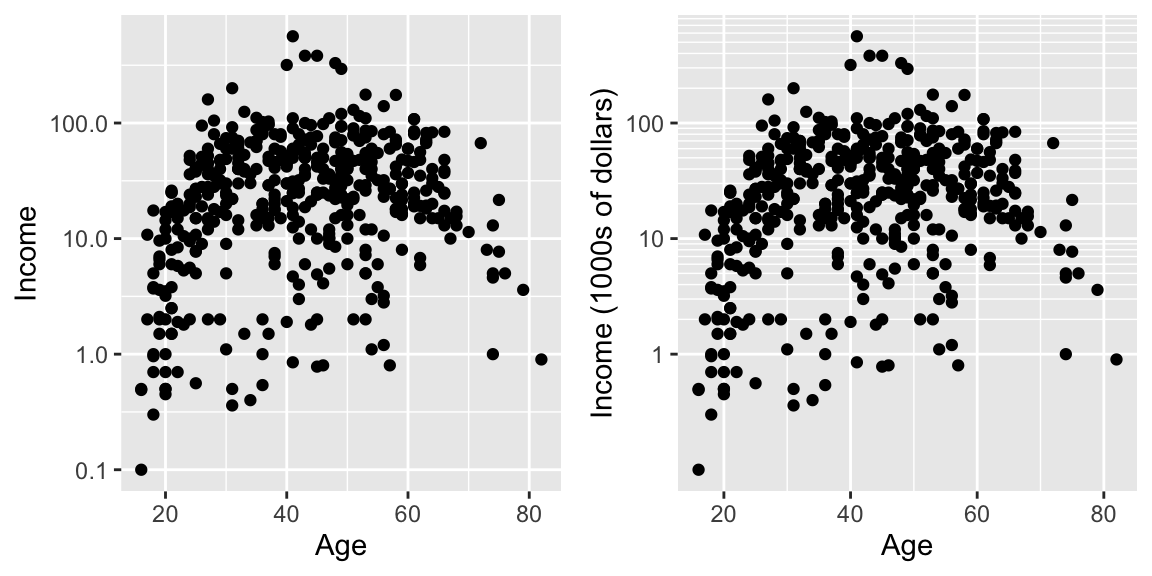While it is certainly possible to specify everything explicitly, I prefer to have a little bit of code to define the minor breaks where all I have to specify is the minimum and maximum order of magnitudes to specify. In the following code, that is the 0:2 components. In the minor breaks, the by=1 or by=2 specifies if we should have 9 or 4 minor breaks per major break.

cowplot::plot_grid(
ggplot(ACS, aes(x=Age, y=Income)) +
geom_point() +
scale_y_log10(
breaks = 10^(0:2),
minor  = outer(seq(0,10,by=1), 10^(0:2)) %>% as.vector()
) +
ylab('Income (1000s of dollars)'),

ggplot(ACS, aes(x=Age, y=Income)) +
geom_point() +
scale_y_log10(
breaks = 10^(0:2),
minor  = outer(seq(0,10,by=2), 10^(0:2)) %>% as.vector()
) +
ylab('Income (1000s of dollars)')
)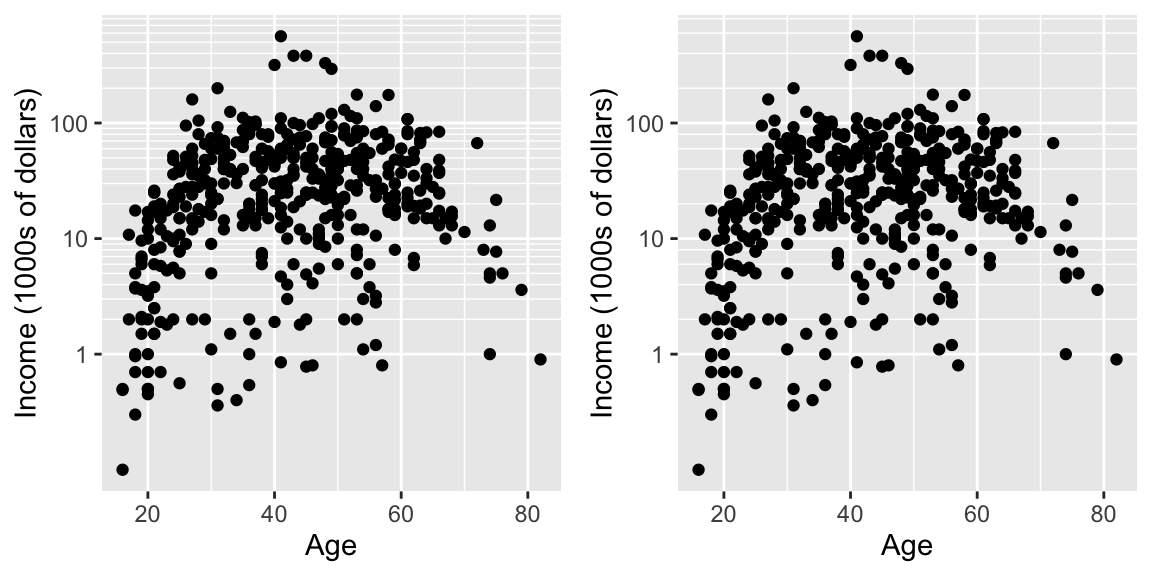Now the y-axis is in the original units (thousands of dollars) but we’d like to customize the minor tick marks. Lets define the major break points (the white lines that have numerical labels) to be at 1,10,100 thousand dollars in salary. Likewise we will tell ggplot2 to set minor break points at 1 to 10 thousand dollars (with steps of 1 thousand dollars) and then 10 thousand to 100 thousand but with step sizes of 10 thousand, and finally minor breaks above 100 thousand being in steps of 100 thousand.

## 14.3 Themes

Many fine-tuning settings in ggplot2 can be manipulated using the theme() function. I’ve used it previously to move the legend position, but there are many other options.

p1 <- ggplot(ChickWeight, aes(x=Time, y=weight, colour=Diet, group=Chick)) +
geom_line() + labs(title='Chick Weight: Birth to 21 days')

# Two common examples of things to change
cowplot::plot_grid(nrow=2,
p1 + theme(plot.title = element_text(hjust = 0.5, size=25)),
p1 + theme(legend.position = 'bottom')                 # legend to bottom
)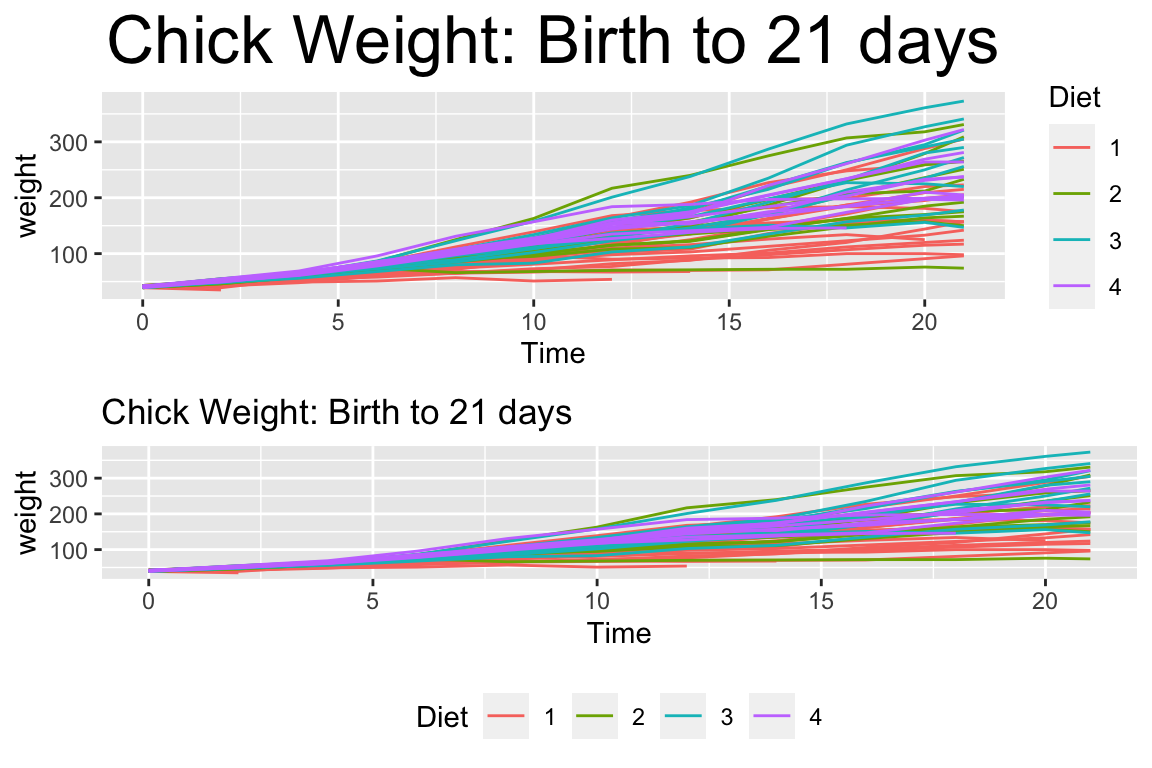There are many things to tweak using the theme() command and to get a better idea of what is possible, I recommend visiting the ggplot2 web page documentation and examples. Notably, one thing that is NOT changed using the theme command is the color scales.

A great deal of thought went into the default settings of ggplot2 to maximize the visual clarity of the graphs. However some people believe the defaults for many of the tiny graphical settings are poor. You can modify each of these but it is often easier to modify them all at once by selecting a different theme. The ggplot2 package includes several, theme_bw(), and theme_minimal() being the two that I use most often. Other packages, such as cowplot and ggthemes, have a bunch of other themes that you can select from. Below are a few examples:

Rmisc::multiplot( cols = 2,
p1 + theme_bw()      + labs(title='theme_bw'), # Black and white
p1 + theme_minimal() + labs(title='theme_minimal'),
p1 + theme_dark()    + labs(title='theme_dark'),
p1 + theme_light()   + labs(title='theme_light')
)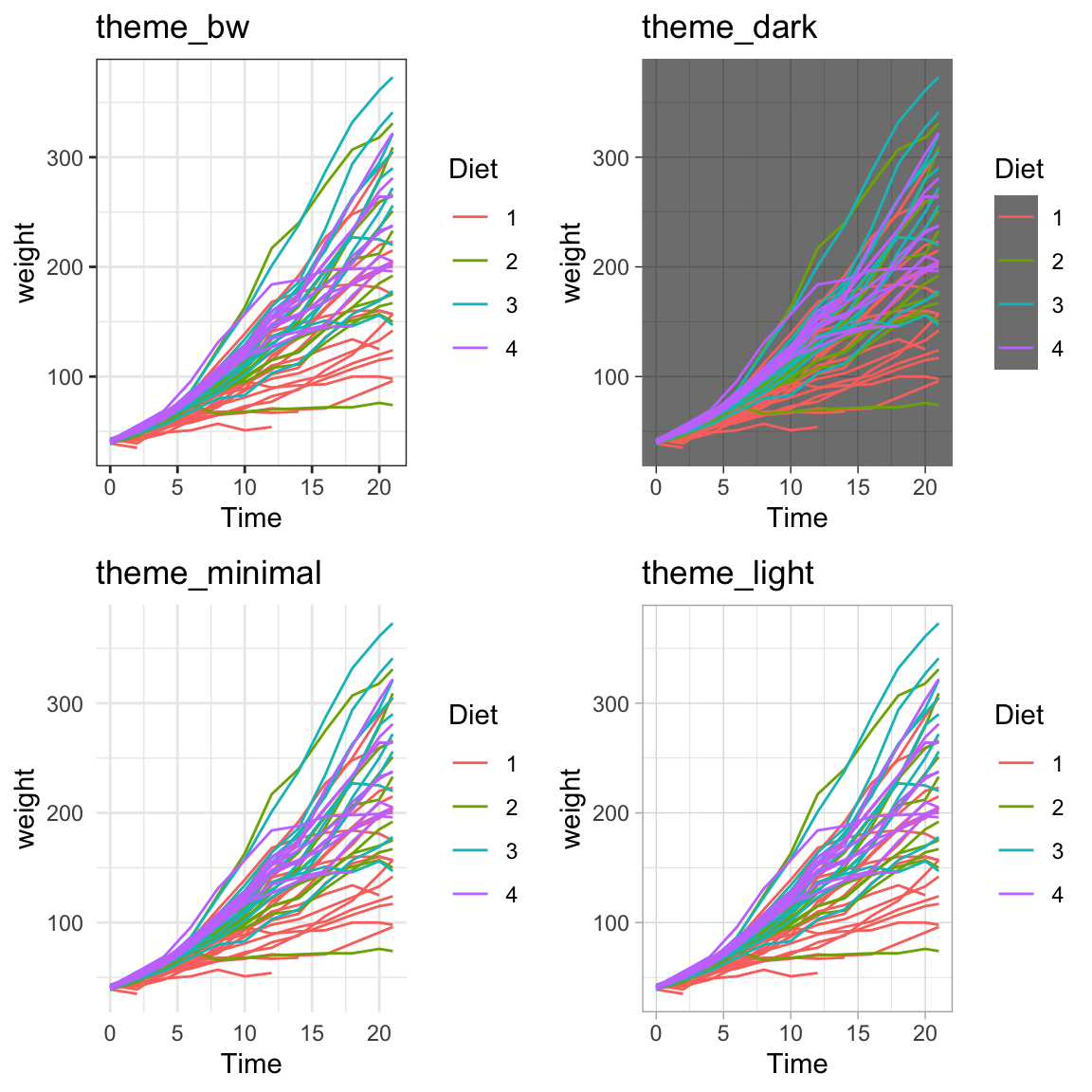Rmisc::multiplot( cols = 2,
p1 + cowplot::theme_cowplot() + labs(title='cowplot::theme_cowplot()'),
p1 + cowplot::theme_minimal_grid() + labs(title='cowplot::theme_minimial_grid'),
p1 + ggthemes::theme_stata() + labs(title='ggthemes::theme_stata()'),
p1 + ggthemes::theme_tufte() + labs(title='ggthemes::theme_tufte()'),
p1 + ggthemes::theme_economist() + labs(title='ggthemes::theme_economist()'),
p1 + ggthemes::theme_fivethirtyeight() + labs(title='ggthemes::theme_fivethirtyeight()'),
p1 + ggthemes::theme_excel_new() + labs(title='ggthemes::theme_excel_new()'),
p1 + ggthemes::theme_gdocs() + labs(title='ggthemes::theme_gdocs()')
)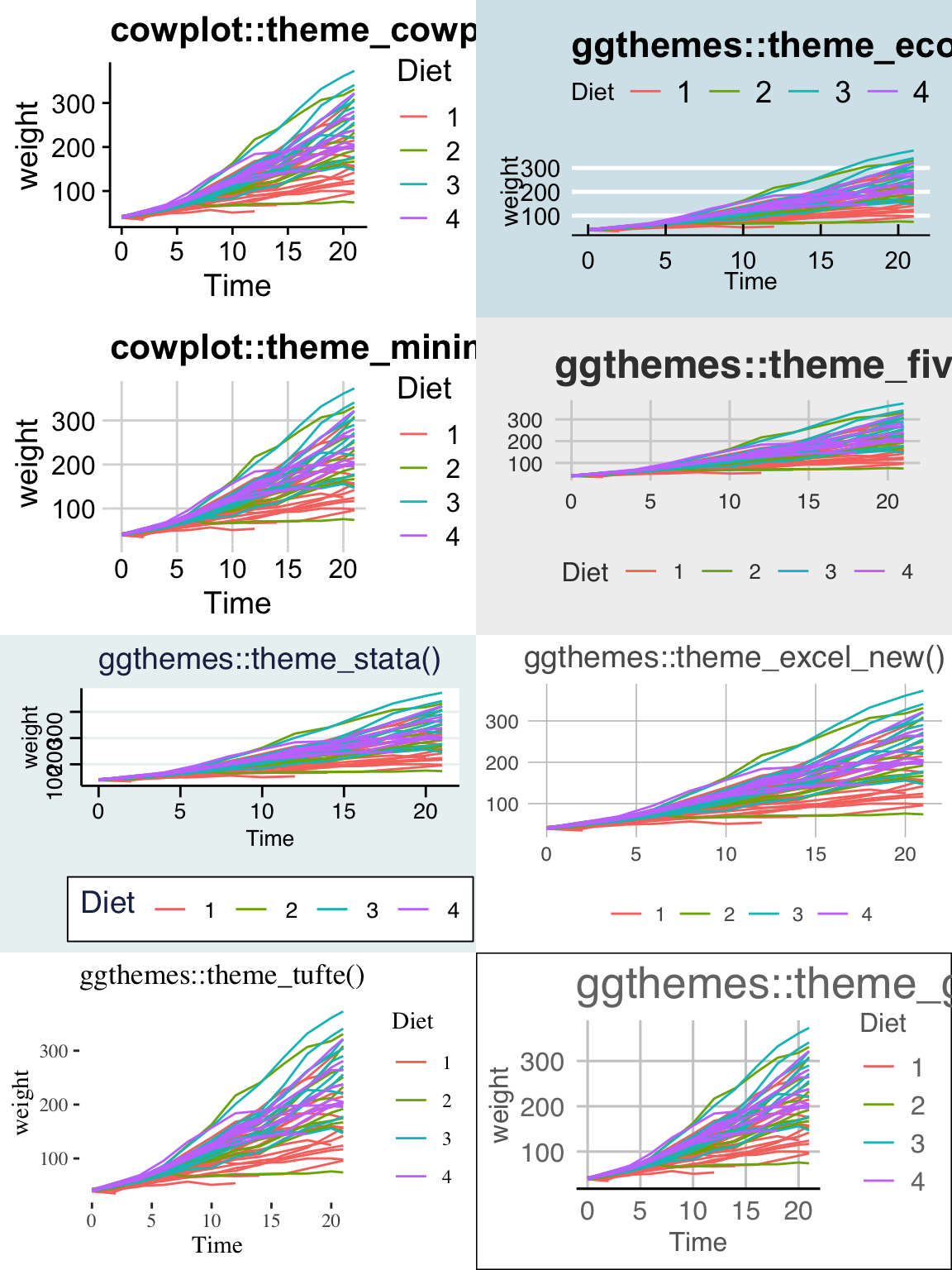Finally, we might want to select a theme for all subsequent plots or modify a specific aspect of the theme.

Command Result
theme_set( theme_bw() ) Set the default theme to be the theme_bw() theme.
theme_update( ... ) Update the current default them.

This will allow you to set the graphing options at the start of your Rmarkdown/R-script document. However the one thing it does not do is allow you to change the default color themes (we still have to do for each graph).

## 14.4 Mathematical Notation

It would be nice to be able to include mathematical formula and notation on plot axes, titles, and text annotation. R plotting has a notation scheme which it calls expressions. You can learn more about how R expressions are defined by looking at the plotmath help help page. They are similar to LaTeX but different enough that it can be frustrating to use. It is particularly difficult to mix character strings and math symbols. I recommend not bothering to learn R expressions, but instead learn LaTeX and use the R package latex2exp that converts character strings written in LaTeX to be converted into R’s expressions.

LaTeX is an extremely common typesetting program for mathematics and is widely used. The key idea is that $ will open/close the LaTeX mode and within LaTeX mode, using the backslash represents that something special should be done. For example, just typing $alpha$ produces $$alpha$$, but putting a backslash in front means that we should interpret it as the greek letter alpha. So in LaTeX, $\alpha$ is rendered as $$\alpha$$. We’ve already seen an introduction to LaTeX in the Rmarkdown Tricks chapter. However, because I need to write character strings with LaTeX syntax, and R also uses the backslash to represent special characters, then to get the backslash into the character string, we actually need to do the same double backslash trick we did in the string manipulations using regular expressions section. seed <- 7867 N <- 20 data <- data.frame(x=runif(N, 1, 10)) %>% # create a data set to work with mutate(y = 12 - 1*x + rnorm(N, sd=1)) # with columns x, y model <- lm( y ~ x, data=data) # Fit a regression model data <- data %>% # save the regression line yhat points mutate(fit=fitted(model)) ggplot(data, aes(x=x)) + geom_point(aes(y=y)) + geom_line(aes(y=fit), color='blue') + annotate('text', x=9, y=9.5, label=latex2exp::TeX('$\\hat{\\rho}$= 0.916') ) + # always double \\ labs( x=latex2exp::TeX('X-axis$\\alpha$'), y=latex2exp::TeX('Y:$\\log_{10}$(Income)'), title=latex2exp::TeX('Linear Models use:$\\hat{\\beta} = (X^TX)^{-1}X^Ty$')) ## Warning in is.na(x): is.na() applied to non-(list or vector) of type ## 'expression'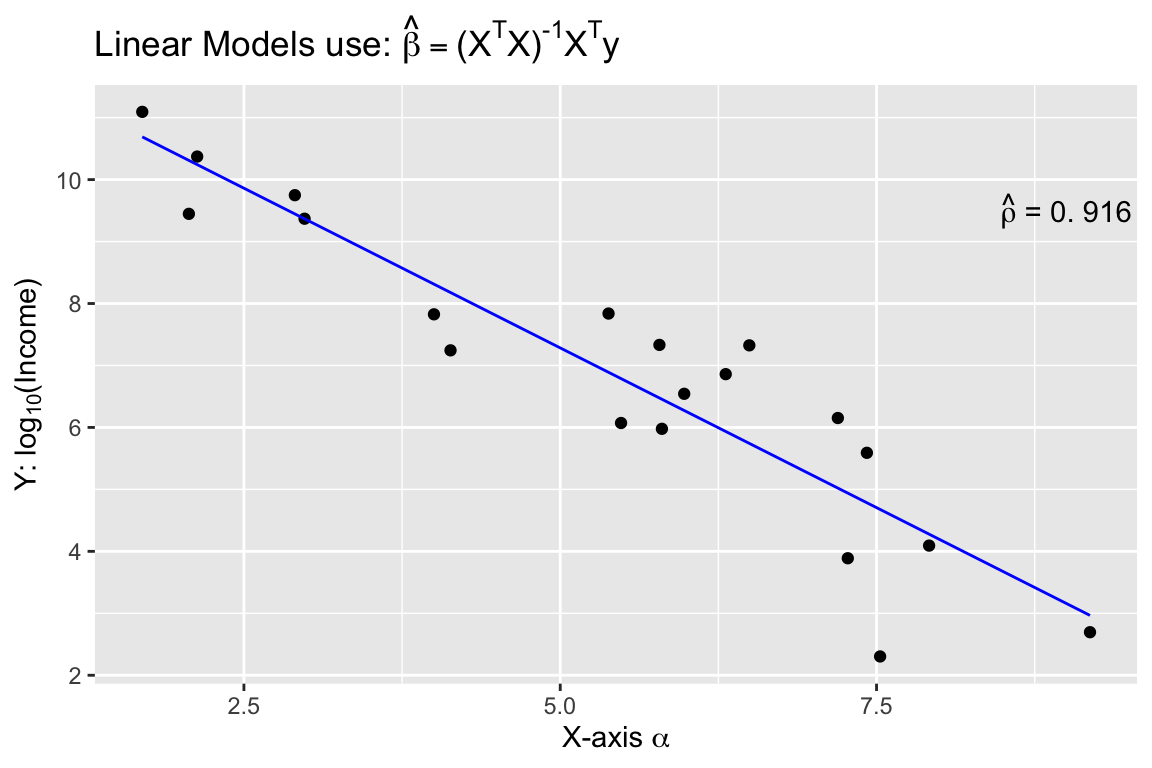The warning message that is produced is coming from ggplot and I haven’t figured out how to avoid it. Because it is giving us the graph we want, I’m just going to ignore the error for now. One issue is how to add expression to a data frame. Unfortunately, neither data.frame nor tibble will allow a column of expressions, so instead we have store it as a character string. Below, we create three character strings using standard LaTeX syntax, and then convert it to a character string that represents the R expression. Finally, in ggplot, we tell the geom_text layer to parse the label and interpret it as an R expression. foo <- data.frame( x=c(1,2,3), y=c(2,2,2) ) %>% mutate( label1 = paste('$\\alpha\$ = ', x) ) %>%       # label is a TeX character string
mutate( label2 = latex2exp::TeX(label1, output = 'character') )  # label2 is an expression character string

ggplot(foo, aes(x=x, y=y) ) +
geom_label( aes(label=label2), parse=TRUE )   # parse=TRUE forces an expression interpretation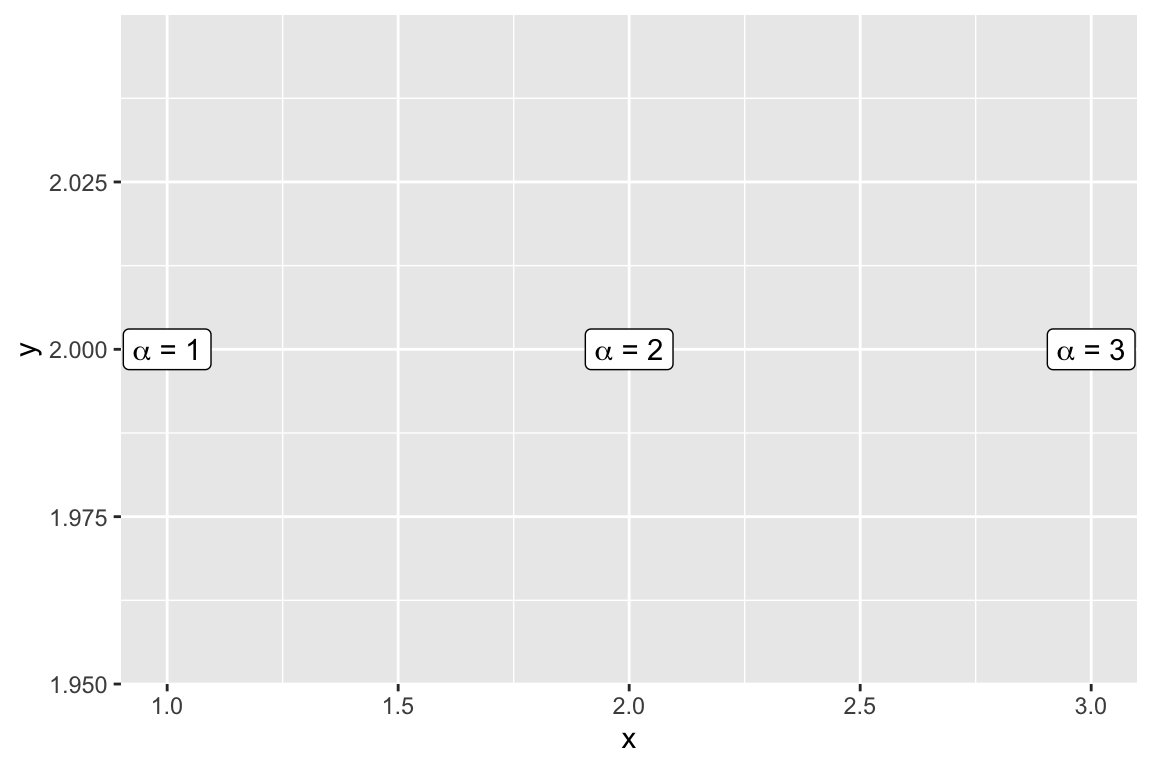## 14.5 Interactive plots with plotly

Plotly is technical computing company that specializes in data visualization. They have created an open source JavaScript library to produce graphs, which is confusingly referred to as plotly. Because plotly is JavaScript, and RStudio’s Viewer pane is actually a web browser, it easily provides interactive abilities in RStudios Viewer pane.

A good tutorial book about using plotly was written by Carson Sievert.

The simple version is that you can take a ggplot graph and pipe it into the ggplotly and it will be rendered into an interactive version of the graph.

P1 <- trees %>%
ggplot(aes(y=Volume, x=Height)) +
geom_point()

P1 %>% plotly::ggplotly()

We can use the widgets to zoom in and out of the graph. Notice that as we hover over the point, it tells us the x/y coordinates. To add information to the hover-text, we just need to add a text aesthetic mapping to the plot.

P1 <- trees %>%
mutate(Obs_ID = row_number()) %>%
ggplot(aes(y=Volume, x=Height,
text=paste('Girth: ', Girth,   '\n',          # add some extra text
'Obs #: ', Obs_ID,  sep=''))) +    # to the hover information
geom_point()

P1 %>% ggplotly() 

## 14.6 Exercises

1. The infmort data set from the package faraway gives the infant mortality rate for a variety of countries. The information is relatively out of date (from 1970s?), but will be fun to graph. Visualize the data using by creating scatter plots of mortality vs income while faceting using region and setting color by oil export status. Utilize a $$\log_{10}$$ transformation for both mortality and income axes. This can be done either by doing the transformation inside the aes() command or by utilizing the scale_x_log10() or scale_y_log10() layers. The critical difference is if the scales are on the original vs log transformed scale. Experiment with both and see which you prefer.

1. The rownames() of the table gives the country names and you should create a new column that contains the country names. *rownames
2. Create scatter plots with the log10() transformation inside the aes() command.
3. Create the scatter plots using the scale_x_log10() and scale_y_log10(). Set the major and minor breaks to be useful and aesthetically pleasing. Comment on which version you find easier to read.
4. The package ggrepel contains functions geom_text_repel() and geom_label_repel() that mimic the basic geom_text() and geom_label() functions in ggplot2, but work to make sure the labels don’t overlap. Select 10-15 countries to label and do so using the geom_text_repel() function.
2. Using the datasets::trees data, complete the following:

1. Create a regression model for $$y=$$ Volume as a function of $$x=$$ Height.

2. Using the summary command, get the y-intercept and slope of the regression line.

3. Using ggplot2, create a scatter plot of Volume vs Height.

4. Create a nice white filled rectangle to add text information to using by adding the following annotation layer.

annotate('rect', xmin=65, xmax=75, ymin=60, ymax=74,
fill='white', color='black') +
5. Add some annotation text to write the equation of the line $$\hat{y}_i = -87.12 + 1.54 * x_i$$ in the text area.

6. Add annotation to add $$R^2 = 0.358$$

7. Add the regression line in red. The most convenient layer function to uses is geom_abline(). It appears that the annotate doesn’t work with geom_abline() so you’ll have to call it directly.

3. In datasets::Titanic table summarizes the survival of passengers aboard the ocean liner Titanic. It includes information about passenger class, sex, and age (adult or child). Create a bar graph showing the number of individuals that survived based on the passenger Class, Sex, and Age variable information. You’ll need to use faceting and/or color to get all four variables on the same graph. Make sure that differences in survival among different classes of children are perceivable. Unfortunately, the data is stored as a table and to expand it to a data frame, the following code can be used.

Titanic <- Titanic %>% as.data.frame()
1. Make this graph using the default theme. If you use color to denote survivorship, modify the color scheme so that a cold color denotes death.
2. Make this graph using the theme_bw() theme.
3. Make this graph using the cowplot::theme_minimal_hgrid() theme.
4. Why would it be beneficial to drop the vertical grid lines?